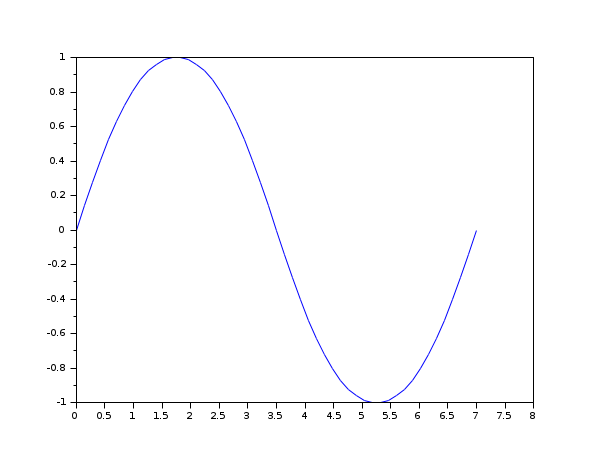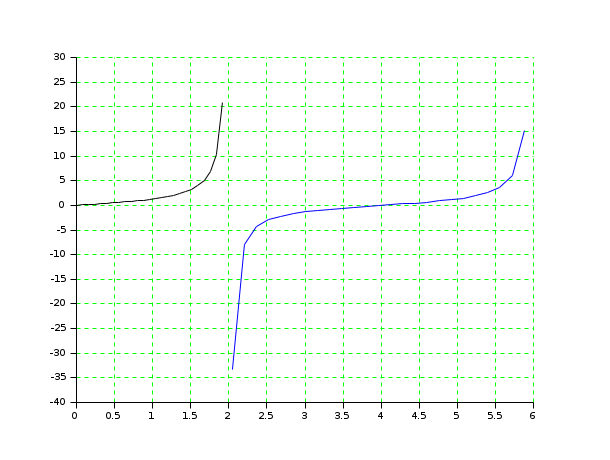Scilab Home page | Wiki | Bug tracker | Forge | Mailing list archives | ATOMS | File exchange
Scilab 5.4.1
Change language to: Français - Português - 日本語 - Русский

Please note that the recommended version of Scilab is 6.1.1. This page might be outdated.
See the recommended documentation of this function

Scilab help >> Special Functions > %sn

# %sn

Jacobi's elliptic function

### Calling Sequence

`[y]=%sn(x,m)`

### Arguments

x

a point inside the fundamental rectangle defined by the elliptic integral; `x` is a vector of complex numbers

m

parameter of the elliptic integral (`0<m<1`)

y

result

### Description

Jacobi 's sn elliptic function with parameter `m`: the inverse of the elliptic integral for the parameter `m`.

The amplitude am is computed in fortran and the addition formulas for elliptic functions are applied

### Examples

```m=0.36;
K=%k(m);
P=4*K; //Real period
real_val=0:(P/50):P;
plot(real_val,real(%sn(real_val,m)))``````clf();
m=0.36;
KK=%k(1-m);
Ip=2*KK;
ima_val1=0:(Ip/50):KK-0.001;
ima_val2=(KK+0.05):(Ip/25):(Ip+KK);
z1=%sn(%i*ima_val1,m);
z2=%sn(%i*ima_val2,m);
plot2d([ima_val1',ima_val2'],[imag(z1)',imag(z2)']);
xgrid(3)```